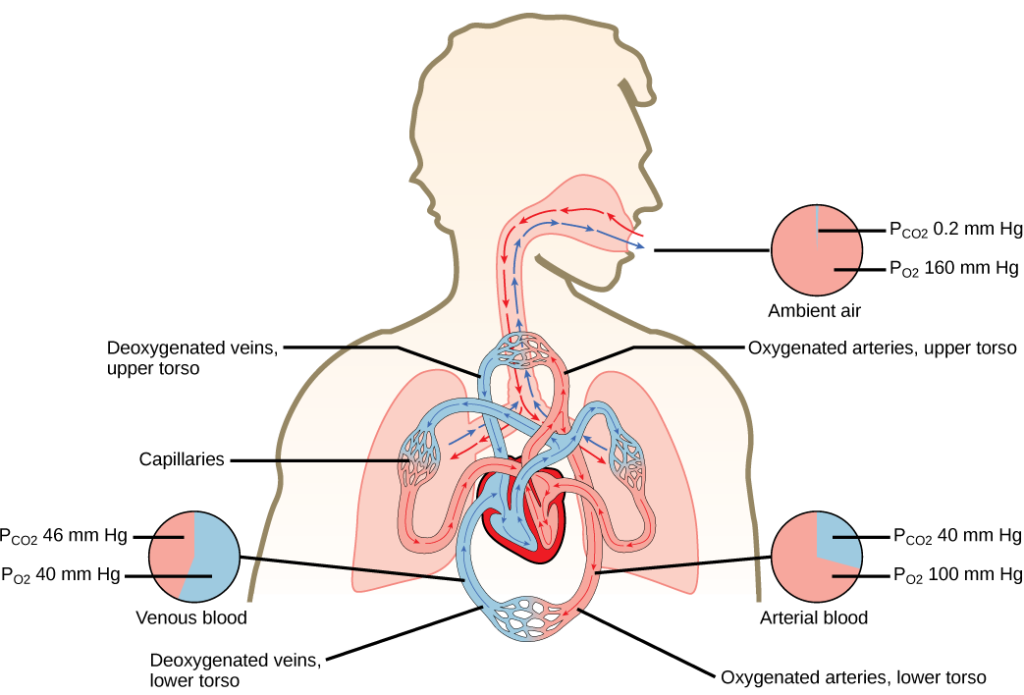## Gas Exchange across the Alveoli

### Learning Outcomes

• Discuss how gases move across the alveoli

In the body, oxygen is used by cells of the body’s tissues and carbon dioxide is produced as a waste product. The ratio of carbon dioxide production to oxygen consumption is the respiratory quotient (RQ). RQ varies between 0.7 and 1.0. If just glucose were used to fuel the body, the RQ would equal one. One mole of carbon dioxide would be produced for every mole of oxygen consumed. Glucose, however, is not the only fuel for the body. Protein and fat are also used as fuels for the body. Because of this, less carbon dioxide is produced than oxygen is consumed and the RQ is, on average, about 0.7 for fat and about 0.8 for protein.

The RQ is used to calculate the partial pressure of oxygen in the alveolar spaces within the lung, the alveolar $\text{P}_{\text{O}_2}$ . Above, the partial pressure of oxygen in the lungs was calculated to be 150 mm Hg. However, lungs never fully deflate with an exhalation; therefore, the inspired air mixes with this residual air and lowers the partial pressure of oxygen within the alveoli. This means that there is a lower concentration of oxygen in the lungs than is found in the air outside the body. Knowing the RQ, the partial pressure of oxygen in the alveoli can be calculated:

$\text{alveolar P}_{\text{O}_2}=\text{inspired P}_{\text{O}_2}-\left(\frac{\text{alveolar P}_{\text{O}_2}}{\text{RQ}}\right)$

With an RQ of 0.8 and a $\text{P}_{\text{CO}_2}$ in the alveoli of 40 mm Hg, the alveolar $\text{P}_{\text{O}_2}$ is equal to:

$\text{alveolar P}_{\text{O}_2}=150\text{ mm Hg}-\left(\frac{40\text{ mm Hg}}{0.8}\right)=\text{mm Hg}$

Notice that this pressure is less than the external air. Therefore, the oxygen will flow from the inspired air in the lung $\left(\text{P}_{\text{O}_2}=150\text{ mm Hg}\right)$ into the bloodstream $\left(\text{P}_{\text{O}_2}=100\text{ mm Hg}\right)$.

In the lungs, oxygen diffuses out of the alveoli and into the capillaries surrounding the alveoli. Oxygen (about 98 percent) binds reversibly to the respiratory pigment hemoglobin found in red blood cells (RBCs). RBCs carry oxygen to the tissues where oxygen dissociates from the hemoglobin and diffuses into the cells of the tissues. More specifically, alveolar $\text{P}_{\text{O}_2}$ is higher in the alveoli $\left(\text{P}_{\text{ALVO}_2}=100\text{ mm Hg}\right)$ than blood $\text{P}_{\text{O}_2}\left(40\text{ mm Hg}\right)$ in the capillaries. Because this pressure gradient exists, oxygen diffuses down its pressure gradient, moving out of the alveoli and entering the blood of the capillaries where O2 binds to hemoglobin. At the same time, alveolar $\text{P}_{\text{CO}_2}$ is lower $\left(\text{P}_{\text{ALVO}_2}=40\text{ mm Hg}\right)$ than blood $\text{P}_{\text{CO}_2}=\left(45\text{ mm Hg}\right)$. CO2 diffuses down its pressure gradient, moving out of the capillaries and entering the alveoli.

Oxygen and carbon dioxide move independently of each other; they diffuse down their own pressure gradients.

As blood leaves the lungs through the pulmonary veins, the venous $\text{P}_{\text{O}_2}$= 100 mm Hg, whereas the venous $\text{P}_{\text{CO}_2}$ = 40 mm Hg. As blood enters the systemic capillaries, the blood will lose oxygen and gain carbon dioxide because of the pressure difference of the tissues and blood. In systemic capillaries, $\text{P}_{\text{O}_2}=100\text{ mm Hg}$, but in the tissue cells, $\text{P}_{\text{O}_2}=40\text{ mm Hg}$. This pressure gradient drives the diffusion of oxygen out of the capillaries and into the tissue cells. At the same time, blood $\text{P}_{\text{CO}_2}=40\text{ mm Hg}$ and systemic tissue $\text{P}_{\text{CO}_2}=45\text{ mm Hg}$. The pressure gradient drives CO2 out of tissue cells and into the capillaries. The blood returning to the lungs through the pulmonary arteries has a venous $\text{P}_{\text{O}_2}=40\text{ mm Hg}$ and a $\text{P}_{\text{CO}_2}=45\text{ mm Hg}$. The blood enters the lung capillaries where the process of exchanging gases between the capillaries and alveoli begins again (Figure 1).Figure 1. The partial pressures of oxygen and carbon dioxide change as blood moves through the body.

### Practice Question

Which of the following statements is false?

1. In the tissues, $\text{P}_{\text{O}_2}$ drops as blood passes from the arteries to the veins, while $\text{P}_{\text{CO}_2}$ increases.
2. Blood travels from the lungs to the heart to body tissues, then back to the heart, then the lungs.
3. Blood travels from the lungs to the heart to body tissues, then back to the lungs, then the heart.
4. $\text{P}_{\text{O}_2}$ is higher in air than in the lungs.

In short, the change in partial pressure from the alveoli to the capillaries drives the oxygen into the tissues and the carbon dioxide into the blood from the tissues. The blood is then transported to the lungs where differences in pressure in the alveoli result in the movement of carbon dioxide out of the blood into the lungs, and oxygen into the blood.

Watch this video to learn how to carry out spirometry.

## Contribute!

Did you have an idea for improving this content? We’d love your input.